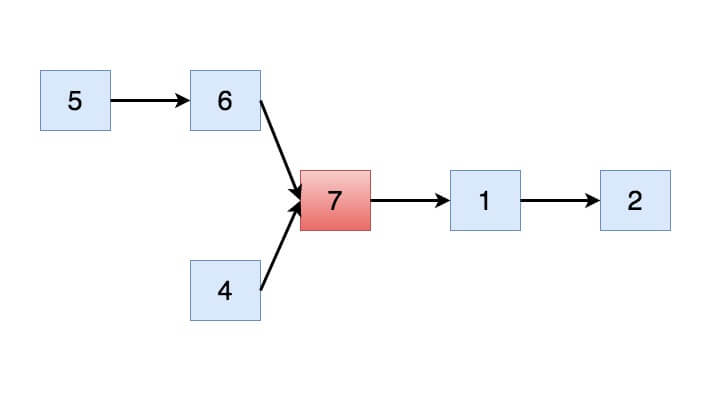# Intersection of two linked lists

In this post, we will see how to find Intersection of two linked lists.

## Problem

Given two singly linked lists, find if two linked lists intersect. If they intersect, find intersection point.## Solution

Here is simple algorithm to find Intersection of two linked lists.

• Find the length of both singly linked lists.
• Find the bigger linked list and iterate up to the difference between two linked list.
• Please note that two linked list will become equal at this step.
• Iterate over both linked list and check if there is any common node, if you find one, return it.
• Else return null.

When you run above program, you will get below output

Intersecting node: 7